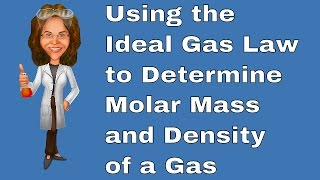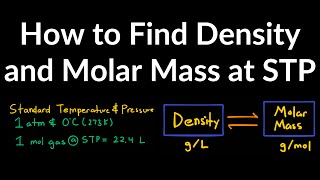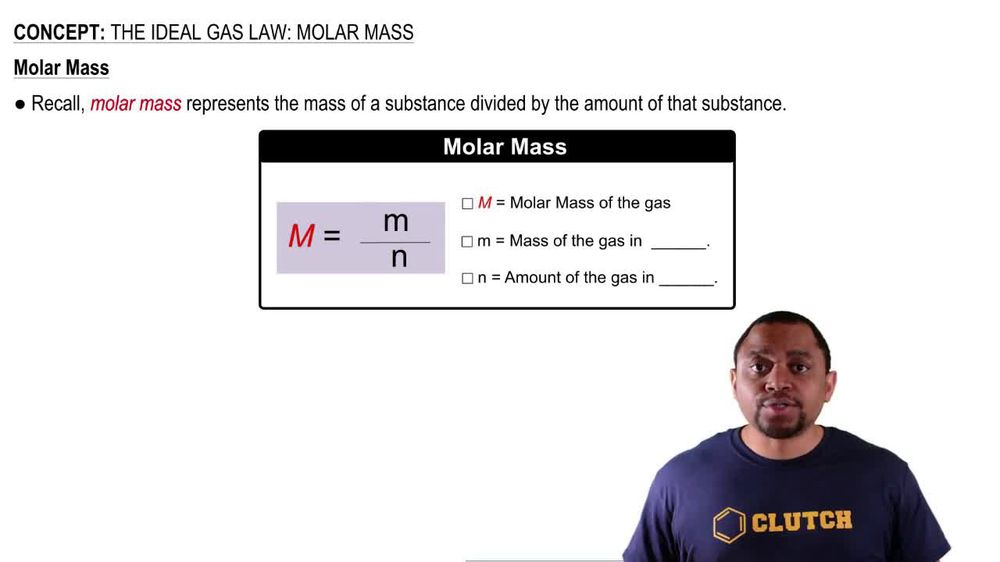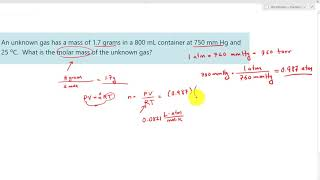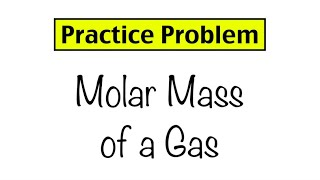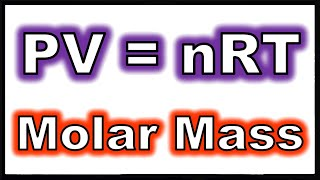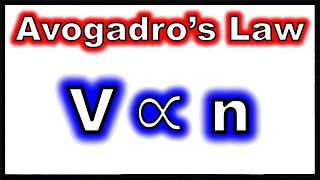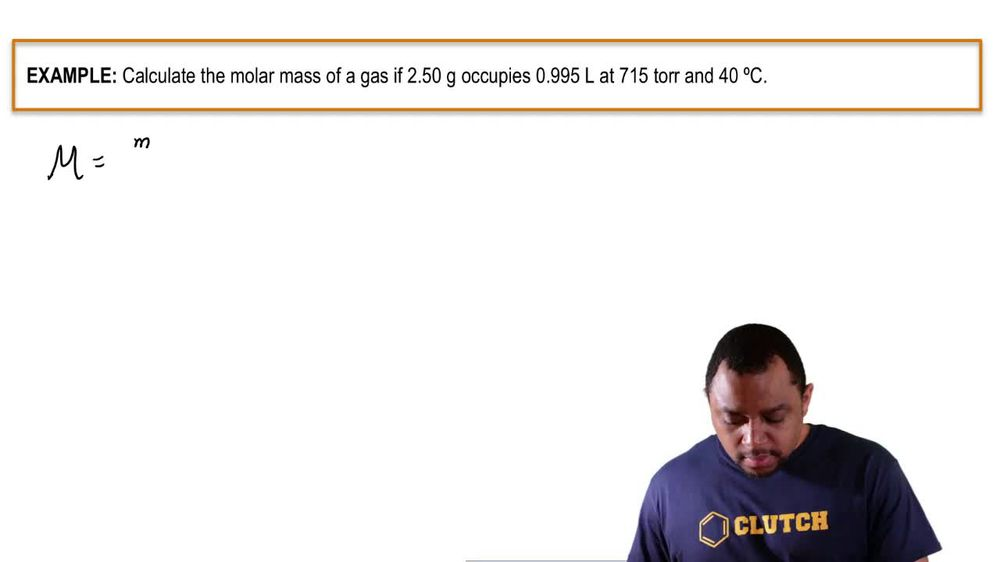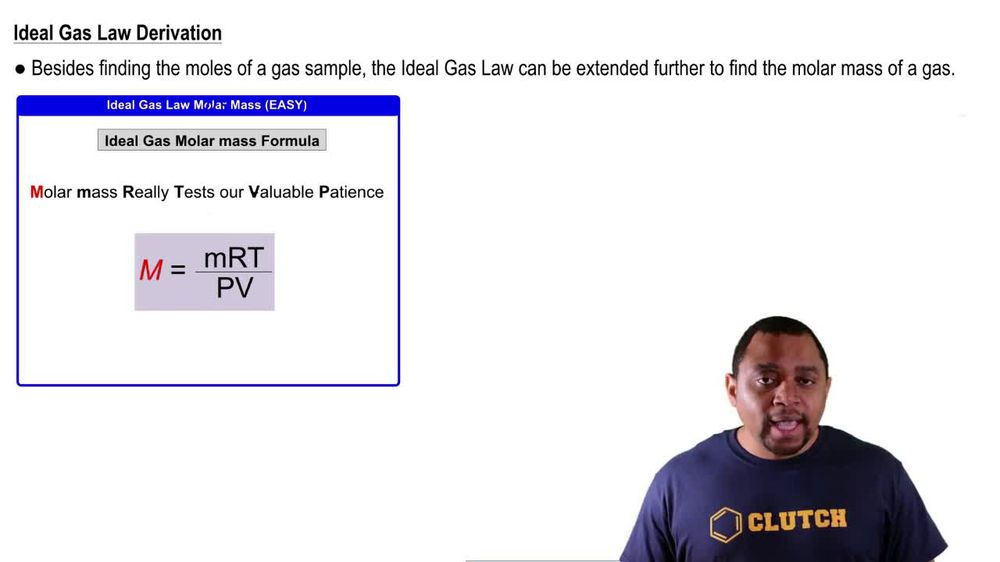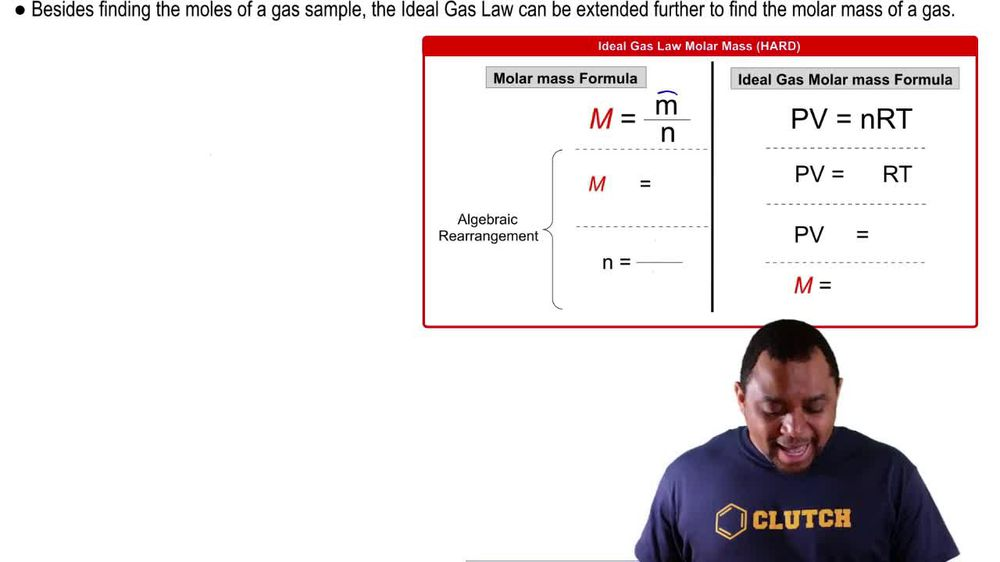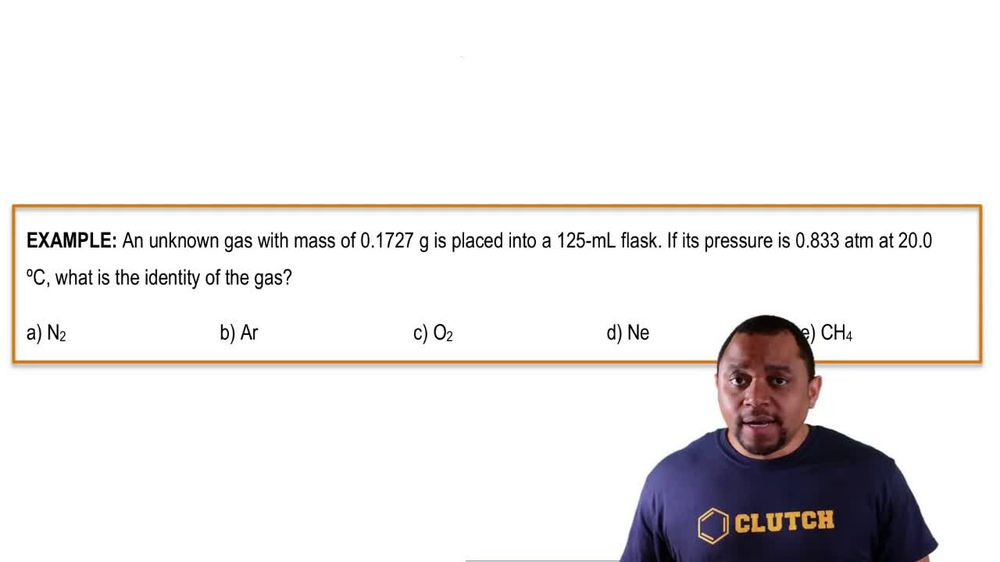Start typing, then use the up and down arrows to select an option from the list.
1. 7. Gases2. The Ideal Gas Law: Molar Mass# The Ideal Gas Law: Molar Mass

by Jules Bruno
101 views
1
1
So for those of you who clicked on this video, let's see how we derived this new version of the ideal gas law. So we start out with the mower mass formula. Remember more mass equals mass m divided by moles. End here we're gonna algebraic rearrange this formula toe isolate, end So first you're gonna multiply both sides by end So now it becomes m Times n equals lower case m toe Isolate our and moles We divide out Moeller Mass So we're gonna say here moles equals mass divided by Mueller Mass going to the ideal gas law formula We can now substitute in for n this value here. So PV equals NRT now becomes PV equals mass over Moeller mass. We need toe isolate our Moeller mass. So what I'm gonna do here is I'm gonna multiply both sides by Mueller Mass Here is the denominator, so I can multiply to get rid of it. And that equals maths times rt finally toe isolate our Mueller mass. We divide both sides by PV and that's how we got that more mass equals MRT over PV again. This is the way we derived it. If your professor wants to show wants you to show the work. But again, it's always easier to remember that Moeller Mass really tests are valuable patients, remembering that will help you remember this version of the ideal gas law.